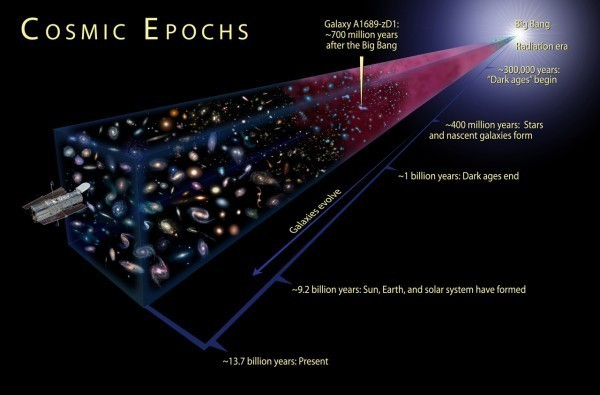# The 5th Dimension

In Dark Energy by Brian Koberlein4 Comments

From measurements of distant supernovae, we now know our universe is not only expanding, but that it is expanding at an ever increasing rate. This cosmic acceleration is driven by what we call dark energy. While we can see the effects of dark energy, and we know it makes up about 68% of our universe, we don’t really know what dark energy actually is. That means while the experimentalists scurry to get more data, the theorists work frantically to explain what’s going on.

In a recent paper in the journal Astronomy and Astrophysics, an interesting theory was proposed to explain dark energy as a quantum effect. The idea is based on an experiment known as the Casimir effect. In quantum mechanics there are energy fluctuations at very small scales. Normally we don’t notice these fluctuations because they average out at larger scales. But you can observe their effect if you constrain the fluctuations. Experimentally this is done by placing two conducting surfaces very close together (on the order of microns). Between these surfaces the fluctuations are limited by the space between the surfaces, but elsewhere the fluctuations aren’t limited. As a result, the conducting surfaces are pulled toward each other, even though there is “nothing” between them.What the Casimir effect demonstrates is that quantum fluctuations in a vacuum can produce a force. So what if dark energy was due to vacuum energy fluctuations? For that to be the case, there would have to be a constrained geometry. But such a constrained geometry is exactly what is proposed in (wait for it) string theory! String theory (or M-theory as it is now known) proposes that there are actually ten spatial dimensions instead of just the three that we know of. These higher dimensions differ from our usual ones in that they are compact, meaning they are folded around themselves. If you think of a straw as a two-dimensional sheet with one “regular” dimension (the length of the straw) and one “compact” dimension (the circumference of the straw), then you have the basic idea.

Now if these compact higher dimensions exist, then quantum fluctuations would be constrained in those dimensions, and that might produce a cosmic Casimir force that looks like dark energy. The catch is that our observations of gravity place a limit on the size of these compact dimensions. This is because gravity is an inverse-square force, meaning that the force of attraction between masses varies by one over their distance squared. If there are higher dimensions, then the inverse-square relation for gravity would break down at the size of those dimensions. Gravity experiments have shown no such effect, so that means that any higher dimensions (if they exist) can be no larger than 85 microns, which is about the width of a human hair.

This is where things get interesting, because if you calculate the effect of these quantum fluctuations in such compact dimensions you find that having 8 compact dimensions (as string theory proposes) doesn’t work. It only works if you allow for just one compact dimension. But if you constrain the universe to just one extra and compact dimension, and you also constrain the fluctuations to exist within the limits of the observable universe, then this model makes two predictions. The first is that dark energy would be constant throughout the universe. It would, in fact, look like a cosmological constant. The second is that this extra compact dimension would have a size of about 35 microns, which means that around 35 microns we should see gravity deviate from the inverse square relation.

Of course all of this could be wrong, but it is an interesting idea with predictions that should be testable in the near future. Sometimes it takes a bit of theory as well as experiments to solve a cosmic mystery.

1.how does the constrained quantum effect in the 5th dimension “leach” into the 3 spacial ones we know?

2.The inverse square relationship concerning gravity (and light) is based on our classical interpretation of our space as having only three dimensions. For example the area of a sphere (in three dimensions) is directly proportional to the square of its radius!! Classical measurements do support this classical interpretation, but only because of our limited measurement capacities. Perhaps a more precise measurement would reveal that this is only an approximate value.

1.Author

Yeah, we’ve tested gravity for higher dimensions, and so far standard gravity still works.

3.Further to the above if our spacial dimensions were four, the inverse square relationship would be an inverse cube relationship. See the area of a four dimensional cube with its relationship to one of its linear dimensions. Again perhaps because of our limited ability to envision dimensions of more than three is what leads us to only be able to measure three.